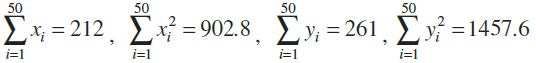The sum and sum of squares corresponding to length x (in cm) and weight y (in gm) of 50 plant products are given below:Which is more varying, the length or weight?

Asked by Abhisek | 1 year ago |  99

##### Solution :-

First we have to calculate Mean for Length x,

$$Mean=\overline{X}=\dfrac{\displaystyle\sum x_i}{n}$$

$$Variance= \dfrac{1}{N^2}[N\displaystyle\sum{f_i}x_i^2-(\displaystyle\sum{f_i}x_i)^2]$$

$$(\dfrac{1}{50^2})[(50\times 902.8)-212^2]$$

$$\dfrac{1}{2500}(45140-44944)$$

$$\dfrac{196}{2500}$$

= 0.0784

Standard deviation σ = $$\sqrt{Variance}$$

$$\sqrt{0.0784}$$  = 0.28

 $$C.V._X=\dfrac{σ}{X}\times 100$$

$$\dfrac{0.28}{4.24}\times 100$$

= 6.603

Now we have to calculate  mean of weight y

$$\overline{Y}=\dfrac{\displaystyle\sum y_i}{n}$$

$$\dfrac{261}{50}=5.22$$

$$Variance= \dfrac{1}{N^2}[N\displaystyle\sum{f_i}y_i^2-(\displaystyle\sum{f_i}y_i)^2]$$

$$(\dfrac{1}{50^2})[(50\times 1457.6)-261^2]$$

$$\dfrac{1}{2500}(72880-68121)$$

$$\dfrac{4759}{2500}$$

= 1.9036

Standard deviation σ = $$\sqrt{Variance}$$

$$\sqrt{1.9036}$$  = 1.37

So, co-efficient of variation of team B,

$$C.V._Y=\dfrac{σ}{X}\times 100$$

$$\dfrac{1.37}{5.22}\times 100$$

= 26.24

Thus, C.V. of weights is greater than the C.V. of lengths.

Therefore, weights vary more than the lengths

Answered by Abhisek | 1 year ago

### Related Questions

#### Find the mean deviation from the mean and from a median of the following distribution

Find the mean deviation from the mean and from a median of the following distribution:

 Marks 0-10 10-20 20-30 30-40 40-50 No. of students 5 8 15 16 6

#### The age distribution of 100 life-insurance policy holders is as follows:

The age distribution of 100 life-insurance policy holders is as follows

 Age (on nearest birthday) 17-19.5 20-25.5 26-35.5 36-40.5 41-50.5 51-55.5 56-60.5 61-70.5 No. of persons 5 16 12 26 14 12 6 5

#### Compute mean deviation from mean of the following distribution:

Compute mean deviation from mean of the following distribution:

 Marks 10-20 20-30 30-40 40-50 50-60 60-70 70-80 80-90 No. of students 8 10 15 25 20 18 9 5

#### Find the mean deviation from the mean for the following data

Find the mean deviation from the mean for the following data:

 Classes 95-105 105-115 115-125 125-135 135-145 145-155 Frequencies 9 13 16 26 30 12